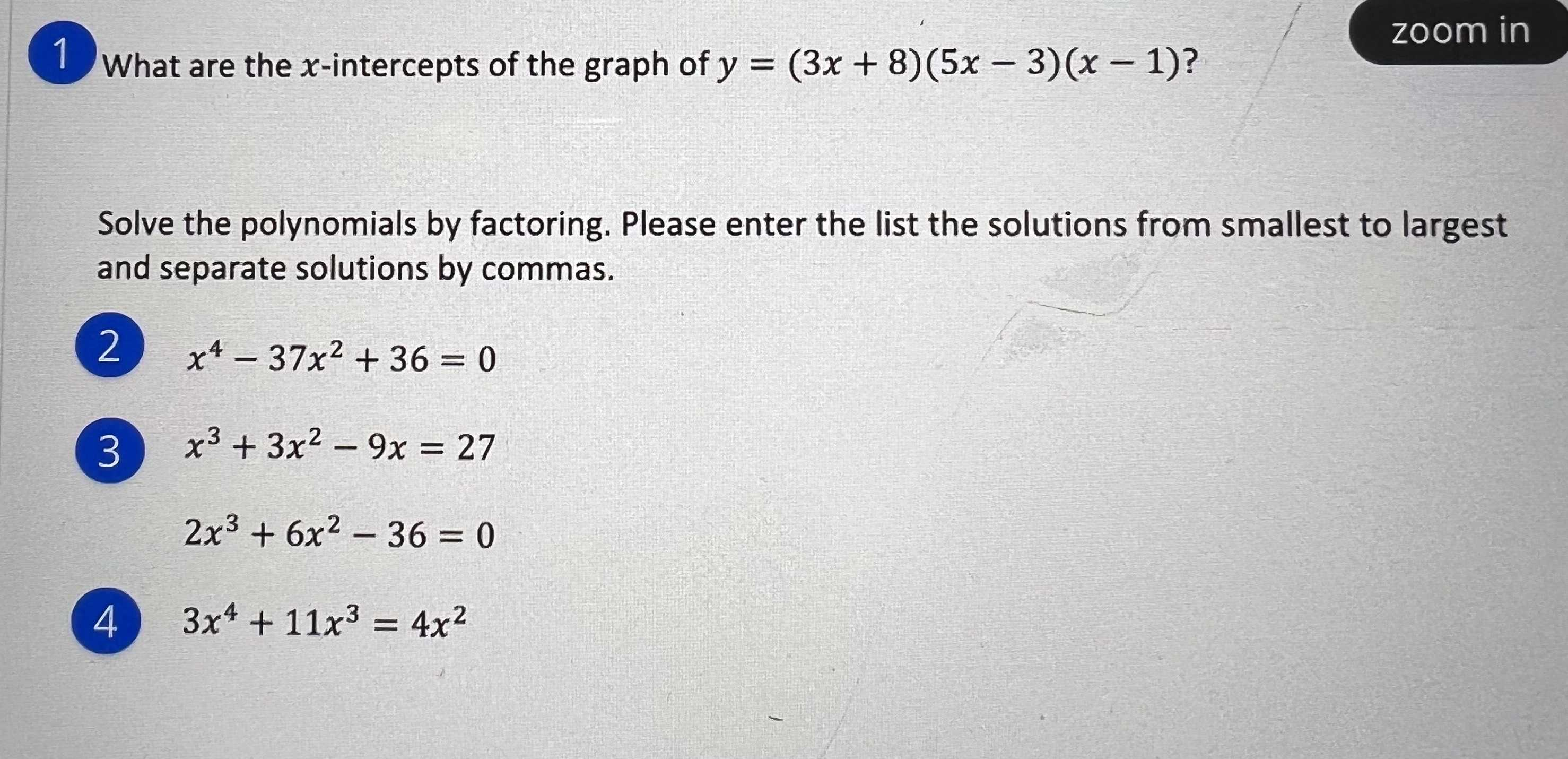### ¿Todavía tienes preguntas de matemáticas?

Pregunte a nuestros tutores expertos
Algebra
Pregunta(1) What are the $$x$$ -intercepts of the graph of $$y = ( 3 x + 8 ) ( 5 x - 3 ) ( x - 1 )$$ ?

Solve the polynomials by factoring. Please enter the list the solutions from smallest to largest and separate solutions by commas.

(2) $$x ^ { 4 } - 37 x ^ { 2 } + 36 = 0$$

(3) $$x ^ { 3 } + 3 x ^ { 2 } - 9 x = 27$$

$$2 x ^ { 3 } + 6 x ^ { 2 } - 36 = 0$$

(4)  $$3 x ^ { 4 } + 11 x ^ { 3 } = 4 x ^ { 2 }$$

1. When y=0, $$x= -\frac{8}{3},\frac{3}{5},1$$

2.

$$x^4- 37x^2+ 36= 0\\\Rightarrow (x^2- 1)(x^2- 36)= 0\\\Rightarrow (x+ 1)(x- 1)(x+ 6)(x- 6)= 0\\\Rightarrow x= -6,- 1,1,6$$

3.

$$x^3+ 3x^2- 9x= 27\\\Rightarrow x^3+ 3x^2- 9x- 27= 0\\\Rightarrow (x^2- 9)(x+ 3)= 0\\\Rightarrow (x+ 3)^2(x- 3)= 0\\\Rightarrow x= -3,3\\\Rightarrow 2x^3+ 6x^2- 36\ne0$$

So, the system has no solution.

4.

$$3x^4+ 11x^3= 4x^2\\\Rightarrow 3x^4+ 11x^3- 4x^2= 0\\\Rightarrow x^2(3x^2+ 11x- 4)= 0\\\Rightarrow x^2(x+ 4)(3x- 1)= 0\\\Rightarrow x= -4,0,\frac{1}{3}$$

Solución
View full explanation on CameraMath App.